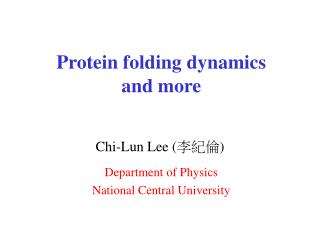DownloadDownload PresentationProtein folding dynamics and more

# Protein folding dynamics and more

Télécharger la présentation## Protein folding dynamics and more

- - - - - - - - - - - - - - - - - - - - - - - - - - - E N D - - - - - - - - - - - - - - - - - - - - - - - - - - -
##### Presentation Transcript

1. Protein folding dynamics and more Chi-Lun Lee (李紀倫) Department of Physics National Central University

2. For a single domain globular protein (~100 amid acid residues), its diameter ~ several nanometers and molecular mass ~ 10000 daltons (compact structure)

3. Introduction Modeling for folding kinetics • N = 100 # of amino acid residues (for a single domain protein) • n = 10 # of allowed conformations for each amino acid residue • For each time only one amino acid residue is allowed to change its state • A single configuration is connected to Nn = 1000 other configurations

4. Concepts from chemical reactions Transition state theory Transition state F DF* Unfolded Folded Reaction coordinate Arrhenius relation : kAB ~ exp(-DF*/T)

5. For complex kinetics, the stories can be much more complicated Statistical energy landscape theory unfolded folded r (order parameter)

6. Energy surface may be rough at times… • Traps from local minima • Non-Arrenhius relation • Non-exponential relaxation • Glassy dynamics

7. Cooperativity in folding Peak in specific heat vs. T c T Resemblance with first order transitions (nucleation)?

8. Theory : to build up and categorize an energy landscape • Defining an order parameter r • Specifying a network • Assigning energy distribution P(E,r) • Projecting the network on the order parameter continuous time random walk (CTRW) Generalized master equation

9. Random energy model Bryngelson and Wolynes, JPC 93, 6902(1989) – e0 ,when the ith residue is in its native state. a Gaussian random variable with mean –e and variance De when the residue is non-native. ei = De – e non-native de – e0 native

10. Random energy model • Another important assumption : random erergy approximation (energies for different configurations are uncorrelated) • This assumption was speculated by the fact that one conformational change often results in the rearrangements of the whole polypeptide chain.

11. Random energy model • For a model protein with N0 native residues, E(N0) is a Gaussian random variable with mean and variance order parameter

12. Random energy model Using a microcanonical ensemble analysis, one can derive expressions for the entropy and therefore the free energy of the system:

13. Kinetics : Metropolis dynamics+CTRW Transition rate between two conformations ( R0 ~ 1 ns ) Folding (or unfolding) kinetics can be treated as random walks on the network (energy landscape) generated from the random energy model

14. Random walks on a network (Markovian) after mapping on r One-dimensional CTRW (non-Markovian) Two major ingredients for CTRW : • Waiting time distribution function • Jumping probabilities

15. can be derived from statistics of the escape rate : And can be derived from the equilibrium condition equilibrium distribution:

16. Let us define probability density that at time t a random walker is at r probability for a random walker to stay at r for at least time t probability to jump from r to r’ in one step after time t

17. Therefore 0 jump 1 jump 2 jumps or Generalized Fokker-Planck equation

18. Results : mean first passage time (MFPT)

19. Results : second moments long-time relaxation Poisson

20. Results : first passage time (FPT) distribution 0 < a < 1 Lévy distribution

21. Power-law exponents for the FPT distribution

22. Locating the folding transition folding transition

23. cf. simulations (Kaya and Chan, JMB 315, 899 (2002))

24. Results : a dynamic ‘phase diagram’ (power-law decay) (exponential decay)

25. A fantasy from the protein folding problem…

26. A ‘toy’ model : Rubik’s cube 3 x 3 x 3 cube : ~ 4x1019 configurations 2 x 2 x 2 cube : 88179840 configurations

27. Metropolis dynamics (on a 2 x 2 x 2 cube) Transition rate between two conformations

28. Monte Carlo simulations

29. Energy : -(total # of patches coinciding with their central-face color)

30. A possible order parameter : depth r (minimal # of steps from the native state) r

31. Free energy

32. Energy fluctuations (T=1.3)

33. A strectched exponential relaxation

34. Two timing in the ‘folding’ process : t1 , t2 Anomalous diffusion Rolling along the order parameter ‘downhill’ : R1 >>1 ‘uphill’ : R1 <<1

35. Summary • Random walks on a complex energy landscape statistical energy landscape theory (possibly non-Markovian) • Local minima (misfolded states) • Exponential nonexponential kinetics • Nonexponential kinetics can happen even for a ‘downhill’ folding process (cf. experimental work by Gruebele et al., PNAS 96, 6031(1999)) Acknowledgment : Jin Wang, George Stell

36. T U t1 , t2 t3 , t4 t1 , t2 U F F • If T is high (e.g., entropy associated with transition state ensemble is small) exponential kinetics likely • If T is low or there is no T nonexponential kinetics

37. Waiting time distribution function long-time scale : power-law decay short-time scale : exponential decay

38. Results : diffusion parameter Lee, Stell, and Wang, JCP 118, 959 (2003)